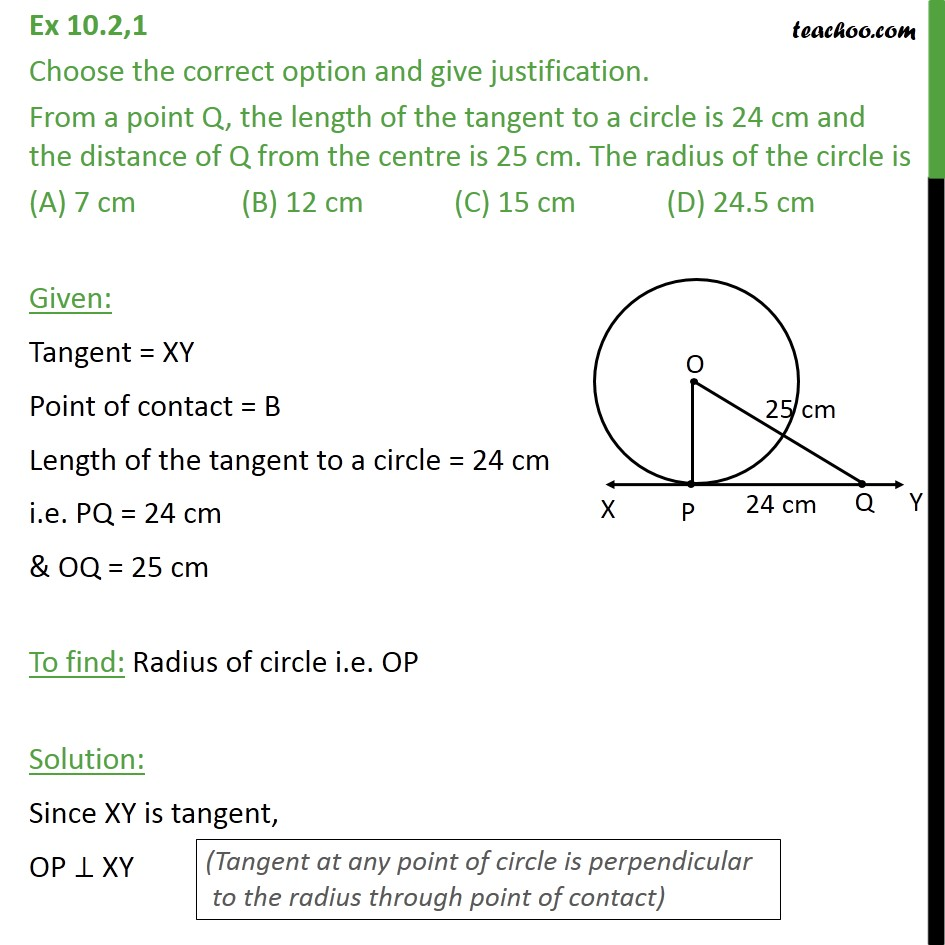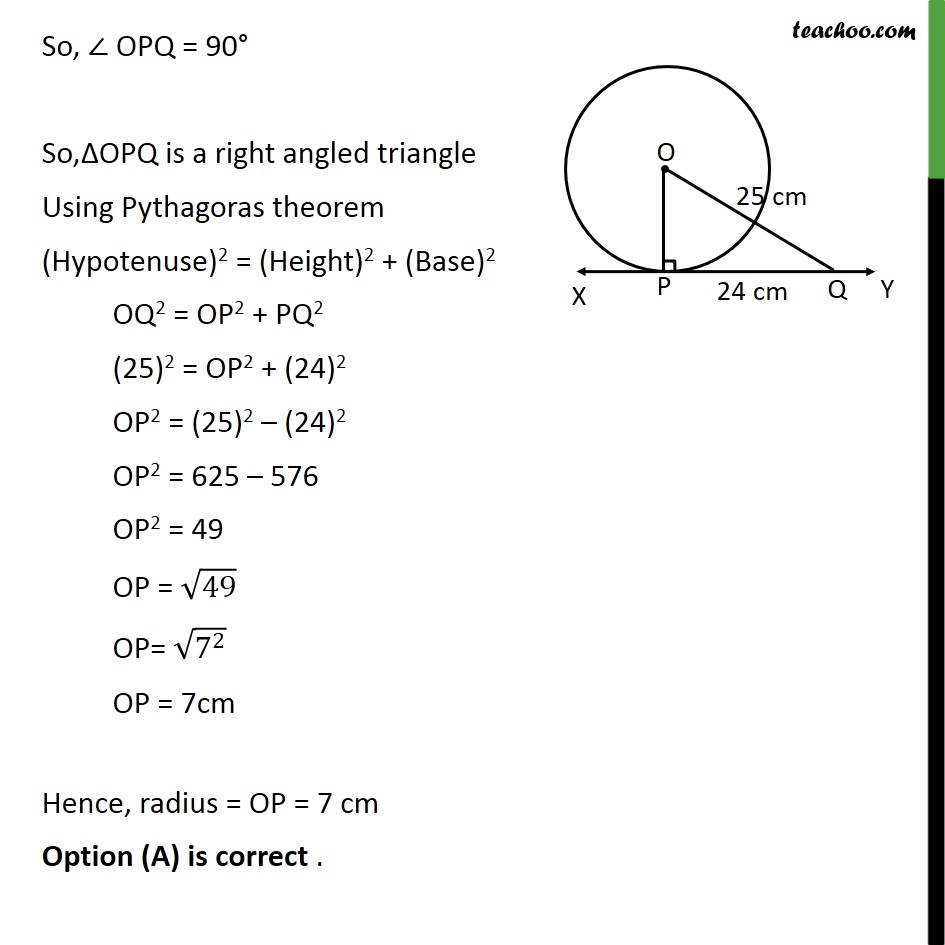Theorem 10.1: Tangent perpendicular to radius (numerical type)Learn in your speed, with individual attention - Teachoo Maths 1-on-1 Class

### Transcript

Ex 10.2,1 Choose the correct option and give justification. From a point Q, the length of the tangent to a circle is 24 cm and the distance of Q from the centre is 25 cm. The radius of the circle is (A) 7 cm (B) 12 cm (C) 15 cm (D) 24.5 cm Given: Tangent = XY Point of contact = B Length of the tangent to a circle = 24 cm i.e. PQ = 24 cm & OQ = 25 cm To find: Radius of circle i.e. OP Solution: Since XY is tangent, OP XY So, OPQ = 90 So, OPQ is a right angled triangle Using Pythagoras theorem (Hypotenuse)2 = (Height)2 + (Base)2 OQ2 = OP2 + PQ2 (25)2 = OP2 + (24)2 OP2 = (25)2 (24)2 OP2 = 625 576 OP2 = 49 OP = 49 OP= (7^2 ) OP = 7cm Hence, radius = OP = 7 cm Option (A) is correct .